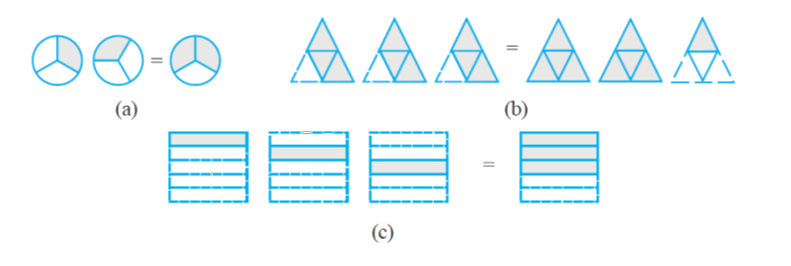# Ex 2.2 Q2 Fractions-and-Decimals-Solutions NCERT Maths Class 7

Go back to  'Ex.2.2'

## Question

Some pictures $$(a)$$ to $$(c)$$ are given below. Tell which of them show:

(i) \begin{align}3 \times \frac{1}{5} = \frac{3}{5}\end{align}

(ii) \begin{align}2 \times \frac{1}{3} = \frac{2}{3}\end{align}

(iii) \begin{align}3 \times \frac{3}{4} = 2\frac{1}{4}\end{align}Video Solution
Fractions And Decimals
Ex 2.2 | Question 2

## Text Solution

What is Known?

Fractions and pictures.

What is unknown?

Matching of fractions with shaded part of the picture.

Reasoning:

Matching can be done by comparing the fractions with the shaded areas of the pictures.

Steps:

(i) \begin{align} 3 \times \frac{1}{5} = \frac{3}{5} \end{align} matches with (c) since, \begin{align} 3 \times \frac{1}{5} = \frac{1}{5} + \frac{1}{5} + \frac{1}{5} = \frac{3}{5} \end{align}

(ii) \begin{align} 2 \times \frac{1}{3} = \frac{2}{3} \end{align}  matches with (a) Since \begin{align} 2 \times \frac{1}{3} = \frac{1}{3} + \frac{1}{3} = \frac{2}{3} \end{align}

(iii) \begin{align} 3 \times \frac{3}{4} = 2\frac{1}{4} \end{align} matches with (b) Since, \begin{align} 3 \times \frac{3}{4} = \frac{3}{4} + \frac{3}{4} + \frac{3}{4} = 2\frac{1}{4} \end{align}

Learn from the best math teachers and top your exams

• Live one on one classroom and doubt clearing
• Practice worksheets in and after class for conceptual clarity
• Personalized curriculum to keep up with school